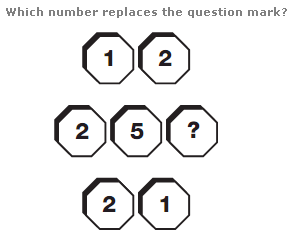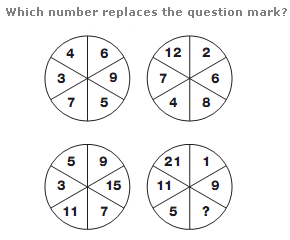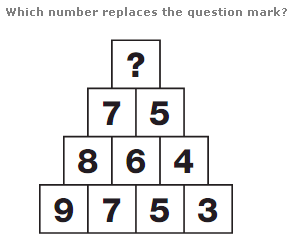# Puzzles - Number puzzles

### Exercise :: Number puzzlesAnswer : 2 Explanation : Reading each row as a whole number, multiply the top 2 digit number by the lower 2 digit number to give the result represented by the middle 3 digit number.Answer : 13 Explanation : Starting with the top circles, multiply each value by 2 and subtract 3, writing the result in the corresponding segment of the lower circle.Answer : 6 Explanation : Numbers in boxes from the second row up equal the average of the 2 numbers in the boxes directly below. Continue to the apex of the triangle.